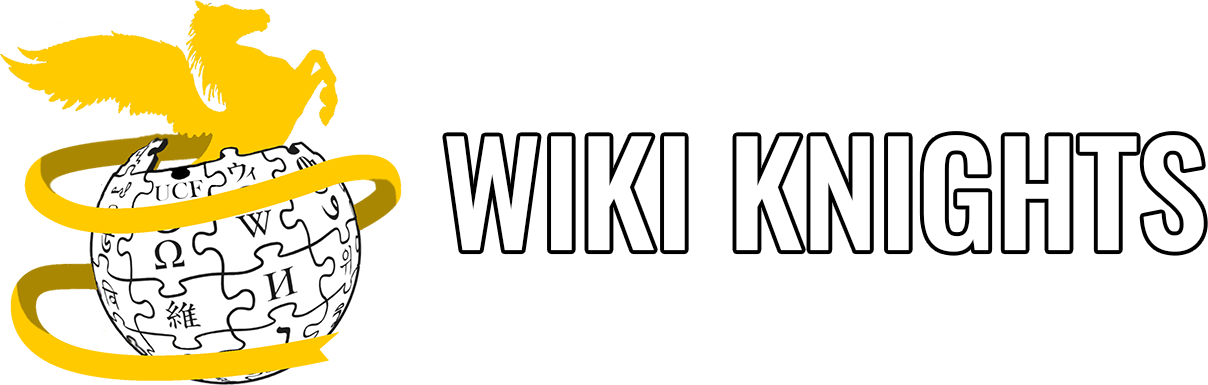# Pre Written Functions (Predefined)

These functions are already defined in the system libraries. So you do not need to code anything, you just need to know in which library it is, what it does and when to use it. Just like a regular written function, predefined functions have a name, datatype parameter and a return type. A good example of a predefined function is the `printf()`. The parameter for the `printf()` is a string and the return type is “void” (but it is omitted) and it is found in the `<stdio.h>` library.

Here are some more fun library functions:

## <stdio.h>

A library full of functions for perfoming input and output.

• printf(): prints to the screen;
• scanf(): scan user input. Here is an example:
``````#include <stdio.h>

int main(void)
{
int a;
printf("Please enter the number 1: ");
scanf("%d", a);

return 0;
}``````

This code will display, if the user has enter the number 1 as requested:

``Please enter the number 1: 1``

## <stdlib.h>

A library with general functions such as: - int rand(): returns a random number; - void srand(int seed): seeds the random number generator used by the function rand; - int abs(int x): returns the absolute value of int x; - void exit(int s): exists the program.

``````#include <stdio.h>
#include <stdlib.h>
#include <time.h>

int main(void)
{
int x = abs(-10);
printf("The absolute value of -10 = %d\n", x);

// seeds the random number generator with the current time
srand(time(NULL));

// generates a number between 1 and 100
int y = 1+rand()%100;

printf("The random number is %d\n", y);

printf("Bye now!\n")

exit(0);

printf("End of the program\n");

return 0;
}``````

This code will display:

``````The absolute value of b = 10
The random number is ~integer~
Bye now!``````

## <math.h> - Math library functions

An assort of mathematical functions. To use them, you must include `<math.h>`. Some of the most used ones are: - double fabs(double x); returns the absolute value of double x; - double pow(double x, double y); return x^y; - double sqrt(double x); returns the square root of x, only works with positive numbers.

Let’s see it how it works in code:

``````#include <stdio.h>
#include <math.h>

int main(void)
{
int a = -3;
int b = fabs(a);

printf("The absolute value of %d is %lf\n", a, b);

// you can also use it inside printf with the appropriate formatter
printf("The value of 2.0 ^ 3 = %lf\n", pow(2, 3));

int c = 25;

printf("The square root of %d is %lf\n", c, sqrt(c));

return 0;
}``````

This code will display:

``````The absolute value of -3 is 3.000000
The value of 2.0 ^ 3 = 8.000000
The square root of 25.000000 is 5.000000``````

You can find more functions from this library here!

## time.h

A library with functions regarding dates and time.

The one we use the most is the `time(NULL)`, which returns the time since the January 1, 1970 (the Epoch), measured in seconds.

``````#include <stdio.h>
#include <stdlib.h>
#include <time.h>

int main(void)
{
time_t seconds;

seconds = time(NULL);
printf("Hours since January 1, 1970 = %ld\n", seconds/3600);

return 0;
}``````

This code will display:

``Hours since January 1, 1970 = 457100``

## ctype.h

This library has functions for mapping characters. - int isalpha(int c);: checks if the passed character is alphabetic; - int tolower(int c);: converts a letter to lowercase; - int toupper(int c);: converts a letter to uppercase.

``````#include <stdio.h>
#include <ctype.h>

int main(void)
{
int a = 'd';
int b = '2';

if(isalpha(a))
{
printf("|%c| is an alphabetic character\n", a);
} else
{
printf("|%c| is not an alphabetic character\n", a);
}

if(isalpha(b))
{
printf("|%c| is an alphabetic character\n", b);
} else
{
printf("|%c| is not an alphabetic character\n", b);
}

char c = 'D';
printf("%c\n", tolower(c));
c = 'e';
printf("%c\n", toupper(c));

return 0;
}``````

This code will display:

``````|d| is an alphabetic character
|2| is not an alphabetic character
d
E``````